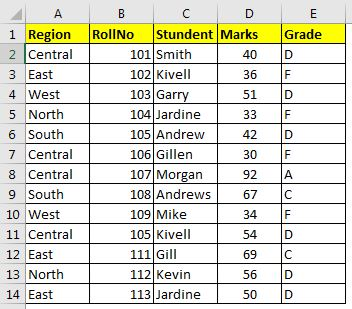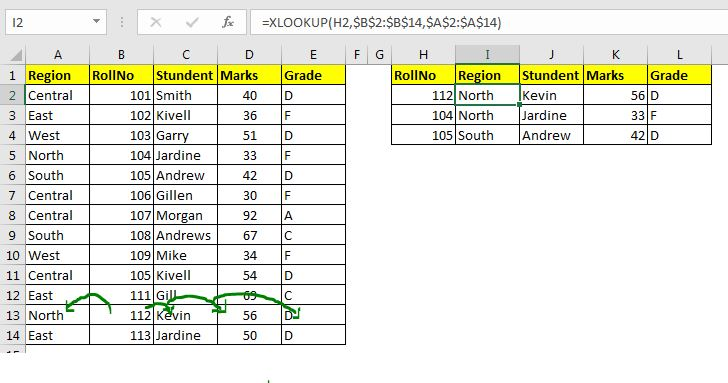# Custom Excel XLOOKUP Function

The XLOOKUP function is exclusive to the insider program of office 365. The LOOKUP function has many functionalities that overcome many of the weaknesses of VLOOKUP and HLOOKUP function but sadly it is not available to us for now. But worry not, we can create an XLOOKUP function that works exactly the same as the upcoming XLOOKUP function MS Excel. We will add functionalities to it one by one.

## VBA code of XLOOKUP function

The below UDF lookup function will solve many problems. Copy it or download xl add-in below file below.

```Function XLOOKUP(lk As Variant, lCol As Range, rCol As Range)

XLOOKUP = WorksheetFunction.Index(rCol, WorksheetFunction.Match(lk, lCol, 0))

End Function
```

Explanation:

The above code is just basic INDEX-MATCH used in VBA. This simplifies a lot of the things that a new user faces. If resolves the complexity of INDEX-MATCH function and uses only three arguments. You can copy it in your excel file, or download the .xlam file below and install it as an add-in excel. If you don't know how to create and use an add-in, click here, it will help you.XLOOKUP Add-In

let's see how it works on excel worksheet.

Syntax of XLOOKUP

 =XLOOKUP(lookup_value, lookup_array, result_array)

lookup_value: This is the value that you want to search in the lookup_array.

lookup_array: It is a one-dimensional range in which you want to search the lookup_value.

result_array:  It is also a one-dimensional range. This is the range from which you want to retrieve the value.

Let's see this XLOOKUP function in action.

### XLOOKUP Examples:Here, I have a data table in excel. Let's explore some functionality using this data table.

Functionality 1. Exact Lookup on the left and right side of the lookup value.

As we know that the Excel VLOOKUP function can't retrieve values from the left of the lookup value. For that, you have to use the complex INDEX-MATCH combination. But not anymore.Assuming that we need to retrieve all the information available in the table of some roll numbers. In that case, you will have to retrieve the region too, which is on the left of roll number column.

Write this formula, I2:

 =XLOOKUP(H2,\$B\$2:\$B\$14,\$A\$2:\$A\$14)

We get the result North for roll number 112. Copy or drag down the formula in below cells to fill them up with the respective regions.

How does it work?

The mechanism is simple. This function looks up the lookup_value in lookup_array and returns the index of a first exact match. Then uses that index to retrieve the value from result_array. This function works perfectly with named ranges.

Similarly use this formula to retrieve the value from each column.Functionality 2. Exact Horizontal Lookup on above and below the lookup value.

The XLOOKUP also works as an exact HLOOKUP function. The HLOOKUP function has the same limitation as the VLOOKUP has. It cant fetch value from above the lookup value. But XLOOKUP not only works as HLOOKUP, it also overcomes that weakness. Let's see how.

Hypothetically, if you want to compare two records. The lookup record you already have. The record you want to compare with is above the lookup_range. Use this formula in that case.

 =XLOOKUP(H7,\$A\$9:\$E\$9,\$A\$2:\$E\$2)

drag down the formula, and you have the entire record of comparing row.Functionality 3. No need for column number and default exact match.

When you use the VLOOKUP function, you have to tell the column number from which you want to fetch the values. For that, you have to count the columns or use some tricks, take the help of other functions. With this UDF XLOOKUP, you don't need to do that.

If you are using VLOOKUP for just fetching some value from one column or to check if a value exists in the column, this is the best solution as per me.

Functionality 4. Replaces INDEX-MATCH, VLOOKUP, HLOOKUP function

For simple tasks, our XLOOKUP function replaces the above-mentioned functions.

Limitations of XLOOKUP:

When it comes to complex formulas, like VLOOKUP with Dynamic Col Index where we VLOOKUP identifies the lookup column with headers, this XLOOKUP will fail.

Another limitation is that if you have to look up multiple random columns or rows from the table, this function will be useless as you have to write this formula again and again. This can be overcome by using named ranges.

For now, we haven't added the approximate functionality, so of course, you can't get the approximate match. We will add that too soon.

If the XLOOKUP function fails to find the lookup value, it will return #VALUE error not #N/A.

So yeah guys, this how you use XLOOKUP to retrieve, search and validate values in excel tables. You can use this user-defined function for a hassle-free lookup on the left or up of the lookup value. If you still have any doubt or any specific requirement related to this function or EXCEL 2010/2013/2016/2019/365 or VBA related query, ask it in the comments section below. You will surely get a reply.

Related Articles:

Create VBA Function to Return Array | To return an array from user defined function, we have to declare it when we name the UDF.

Arrays in Excel Formul|Learn what arrays are in excel.

How to Create User Defined Function through VBA | Learn how to create user-defined functions in Excel

Using a User Defined Function (UDF) from another workbook using VBA in Microsoft Excel | Use the user-defined function in another workbook of Excel

Return error values from user-defined functions using VBA in Microsoft Excel | Learn how you can return error values from a user-defined function

Popular Articles:

Split Excel Sheet Into Multiple Files Based On Column Using VBA | This VBA code split excel sheet base on unique values in a specified column. Download the working file.

Turn Off Warning Messages Using VBA In Microsoft Excel 2016 | To turn off warning messages that interrupt the running VBA code, we use the Application class.

Add And Save New Workbook Using VBA In Microsoft Excel 2016 | To add and save workbooks using VBA we use Workbooks class. Workbooks.Add adds new workbook easily, however...

1.This is very useful and so simple and cool to use....

Is it possible to add, [not_found] functionality in this Function...

Thanks alot...

•Yes, you can encapsulate this formula into if error.
2.